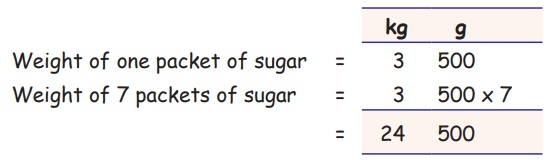Home | | Maths 5th Std | Multiplication and Division in Weight

# Multiplication and Division in Weight

Measurements : Multiplication and Division of Weight

Multiplication

Examples

i. 7 kg 400 g × 37kg 400g × 3 = 22kg 200g

(ii) 52 kg 350 g × 852 kg 350 g × 8 = 418 kg 800 g

Try this

Multiply the following:

a. 7 kg 350 g × 7 = 51kg 450g

b. 9 kg 750 g × 3 = 29kg 250g

c. 9 kg 750 g × 3 = 29kg 250 g

d. 45 kg 800 g × 6 = 274kg 800g

Example

A packet can contain 3 kg 500 g of sugar, how much amount of sugar can 7 such packets contain?

Solution:Weight of 7 packets of sugar = 24 kg 500 g

Division

Examples

Divide the following:

(i) 70 kg 350 g ÷ 770 kg 350 g ÷ 7 = 10 kg 50 g

(ii) 66 g 720 mg ÷ 666 g 720 mg ÷ 6 = 11 g 120 mg

Try this

Divide the following:

a. 7 kg 490 g ÷ 7 = 1kg 070g

b. 35 kg 650 g ÷ 5 = 7kg 130g

c. 6 g 240 mg ÷ 4 = 1g 560mg

d. 150 g 750 mg ÷ 15 = 10g 050mg

Example

How many packets of 3 kg groundnut cookies can be made from 75 kg of groundnut cookies?Solution:

Total amount of groundnut cookies = 75 kg

Weight of 1 packet of groundnut cookies = 3 kg

Number of packets of 3 kg groundnut cookies = 75÷3

= 25 packets

we can separate 75 kg groundnut cookies as 3 kg groundnut cookies in 25 packets.

Tags : Measurements | Term 2 Chapter 4 | 5th Maths , 5th Maths : Term 2 Unit 4 : Measurements
Study Material, Lecturing Notes, Assignment, Reference, Wiki description explanation, brief detail
5th Maths : Term 2 Unit 4 : Measurements : Multiplication and Division in Weight | Measurements | Term 2 Chapter 4 | 5th Maths